### OUTPUT Statement

OUTPUT <OUT=SAS-data-set> keyword=name <…keyword=name> ;

The OUTPUT statement creates an output SAS data set that contains statistics calculated after fitting the model. At least one specification of the form keyword=name is required.

All variables in the original data set are included in the new data set, along with the variables that are created with keyword options in the OUTPUT statement. These new variables contain fitted values and estimated quantiles. If you want to create a SAS data set in a permanent library, you must specify a two-level name. For more information about permanent libraries and SAS data sets, see SAS Language Reference: Concepts.

The following specifications can appear in the OUTPUT statement:

OUT=SAS-data-set

specifies the new data set. By default, the procedure uses the DATAn convention to name the new data set.

keyword=name

specifies the statistics to include in the output data set and gives names to the new variables. Specify a keyword for each desired statistic (see the following list), an equal sign, and the variable to contain the statistic.

The keywords allowed and the statistics they represent are as follows:

LEVERAGE

specifies a variable to indicate leverage points. To include this variable in the OUTPUT data set, you must specify the LEVERAGE option in the MODEL statement. See the section Leverage Point and Outlier Detection for how to define LEVERAGE.

MD

specifies a variable to contain the Mahalanobis distances. See the section Robust Distance for the definition of Mahalanobis distance.

OUTLIER

specifies a variable to indicate outliers. See the section Leverage Point and Outlier Detection for information about how to define OUTLIER.

PMD

specifies a variable to contain the projected Mahalanobis distances. See the section Robust Distance for the definition of projected Mahalanobis distance.

POD

specifies a variable to contain the projected off-plane distances. See the section Robust Distance for the definition of off-plane distance.

PRD

specifies a variable to contain the projected robust MCD Mahalanobis distances. See the section Robust Distance for the definition of projected robust distance.

PREDICTED | P

specifies a variable to contain the estimated responses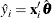RD

specifies a variable to contain the robust MCD Mahalanobis distances. See the section Robust Distance for the definition of robust distance.

RESIDUAL | R

specifies a variable to contain the unstandardized residuals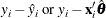SRESIDUAL | SR

specifies a variable to contain the standardized residuals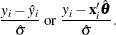By default, the LTS method uses Wscale as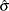for computing the standardized residuals.

STDP

specifies a variable to contain the estimates of the standard errors of the estimated mean responses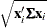where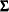denotes the covariance matrix of the parameter estimates. You can request the ODS table of this covariance matrix by using the COVB option of the MODEL statement. The STDP= option is applied to M, S, and MM estimation, but not to LTS estimation.

STDI

specifies a variable to contain the estimates of the standard errors of the individual predicted values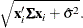The STDI= option is applied to M, S, and MM estimation, but not to LTS estimation.

WEIGHT

specifies a variable to contain the computed final weights.